# Estatísticas de Resumo

• Nota de Estudos
• Rever Tópicos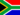No M. 0 0 There are three measures for finding the center of a set of data: the mean, the median and the mode(central tendencies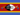Samkeliso R S. 0 0 the mean, meadian and the mode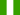Juliet N. 0 0 three measures of central tendercies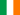Rachel N. 0 0 Summary statistics There are three measures for finding the centre of a set of data: the mean, the median and the mode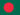Jahidul I. 0 0 summary statistics are used to summarize a set of observations, in order to communicate the largest amount of information as simply as possible. Statisticians commonly try to describe the observations in a measure of location, or central tendency, such as the arithmetic mean a measure of statistical dispersion like the standard deviation a measure of the shape of the distribution like skewness or kurtosis if more than one variable is measured, a measure of statistical dependence such as a correlation coefficientJahidul I. 0 0 what is Standard Deviation ?
• Text Version### Você recebeu uma nova notificação

Clique aqui para visualizar todos eles